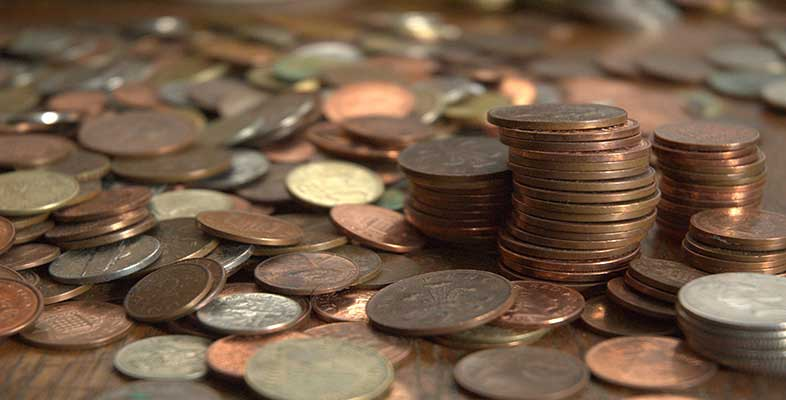### Become an OU studentIntroduction to bookkeeping and accounting

Start this free course now. Just create an account and sign in. Enrol and complete the course for a free statement of participation or digital badge if available.

# 1.4 Fractions

So far we have thought of numbers in terms of their decimal form, e.g., 4.567, but this is not the only way of thinking of, or representing, numbers. A fraction represents a part of something. If you decide to share out something equally between two people, then each receives a half of the total and this is represented by the symbol ½.

A fraction is just the ratio of two numbers: 1/2, 3/5, 12/8, etc. We get the corresponding decimal form 0.5, 0.6, 1.5 respectively by performing division. The top half of a fraction is called the numerator and the bottom half the denominator, i.e., in 4/16, 4 is the numerator and 16 is the denominator. We divide the numerator (the top figure) by the denominator (the bottom figure) to get the decimal form. If, for instance, you use your calculator to divide 4 by 16 you will get 0.25.

A fraction can have many different representations. For example, 4/16, 2/8, and 1/4 all represent the same fraction, one quarter or 0.25. It is customary to write a fraction in the lowest possible terms. That is, to reduce the numerator and denominator as far as possible so that, for example, one quarter is shown as 1/4 rather than 2/8 or 4/16.

If we have a fraction such as 26/39 we need to recognise that the fraction can be reduced by dividing both the denominator and the numerator by the largest number that goes into both exactly. In 26/39 this number is 13 so (26/13) / (39/13) equates to 2/3.

We can perform the basic numerical operations on fractions directly. For example, if we wish to multiply 3/4 by 2/9 then what we are trying to do is to take 3/4 of 2/9, so we form the new fraction: 3/4 x 2/9 = (3 x 2) / (4 x 9) = 6/36 or 1/6 in its simplest form.

In general, we multiply two fractions by forming a new fraction where the new numerator is the result of multiplying together the two numerators, and the new denominator is the result of multiplying together the two denominators.

Addition of fractions is more complicated than multiplication. This can be seen if we try to calculate the sum of 3/5 plus 2/7. The first step is to represent each fraction as the ratio of a pair of numbers with the same denominator. For this example, we multiply the top and bottom of 3/5 by 7, and the top and bottom of 2/7 by 5. The fractions now look like 21/35 and 10/35 and both have the same denominator, which is 35. In this new form we just add the two numerators.

(3/5) + (2/7) = (21/35) + (10/35)

= (21 + 10) / 35

= 31/35

## Activity 6

### Part (a - i)

(a) Convert the following fractions to decimal form (rounded to three decimal places) by dividing the numerator by the denominator on your calculator:

(i) 125/1000

(i) 0.125

(ii) 8/24

(ii) 0.333

(iii) 32/36

(iii) 0.889

### Part (b - i)

(b) Perform the following operations between the fractions given:

(i) 1/2 x 2/3

(i) 1/3

### Part (b - ii)

(ii) 11/34 x 17/19

(ii) 187/646 = 11/38 (if top and bottom both divided by 17)

(iii) 2/5 x 7/11

(iii) 14/55

(iv) 1/2 + 2/3